# Unknown number 16

My number's tens is three times more then ones
My number's ones is twice the number of thousands
and my number's hundreds is half the number of of tens.
I have two ones.
which number am I?

x =  11362

### Step-by-step explanation:

$x=11362=11362$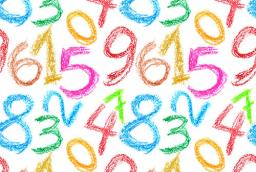Did you find an error or inaccuracy? Feel free to write us. Thank you!## Related math problems and questions:

• Unknown numberI think the number: Its half is 16 bigger than his quarter. I think the number N . ..
• DivisionWhich number in division 16 give 12 and the rest 3?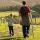Daddy is a 46-year-old, and his son is at the age of 16. When (which year) was/will daddy five times more years than his son?
• Unknown number 22I am a two-digit number. My tens digits are 4. My one digit is three more than my tens. Who am I?
• My fatherMy father has a big farm. 6/8 of it were planted with mango trees, 1/2 of the remainder are guava trees and the rest 10 trees are santol trees. What is the number of all trees?
• Rounding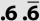Double round number 727, first to tens, then to hundreds. (double rounding)
• Unknown number 5I think of an unknown number. If we enlarge it five times, then subtract 3, and the result decreases by 75%, we get one greater than the number. What number am I thinking of?
• Perpetrator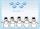A perpetrator is a number that is smaller than the number 80. It is a multiple of five, and it is odd. If we add up the tens and ones of this number, we get the number 8.
• Unknown number 2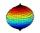I think the number. When he reduces it four times, I'll get 11. What number am I thinking?
• Written numberPlace+values x ten thousands =30 thousands
• DigitsHow many odd four-digit numbers can we create from digits: 0, 3, 5, 6, 7? (a) the figures may be repeated (b) the digits may not be repeated
• NumberDetermine unknown number if you know that difference between five times and triple of number is 42.
• Chocolate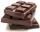How many times do we have to break a chocolate bar composed of 10 × 12 pieces to get the 120 parts?
• Unknown number 24I think the number: a - is the same as the square area that has the 12th circumference. What is this number? b - its half is 7 times bigger than its quarter. Is this the number?
• JakubJakub multiplied 11 times the number 100 by ten and then divided the result ten times by 10. What number did he get?
• Unknown numberSamuel wrote unknown number. Then he had add 200000 to the number and the result multiply by three. When it calculated he was surprised, because the result would have received anyway, if write digit to the end of original number. Find unknown number.
• Brick weightThe brick weighs 3 kg and a half-brick. How much does one brick weigh?